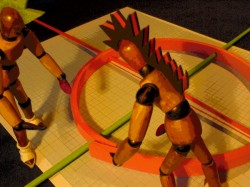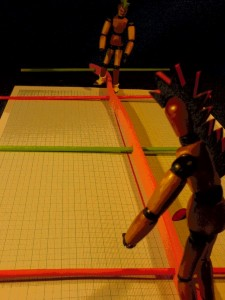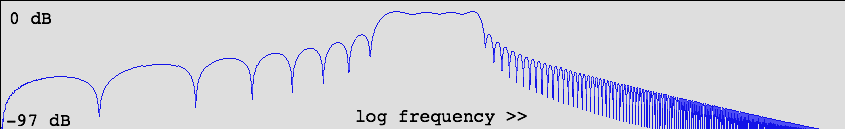# long FIR filters with low latency

minimum-phase filter kernels and partitioned convolution

Previous pages described parametric Fourier filters which can do radical and precise filtering, but which suffer from considerable latency, due to FFT length (25 - 50 ms typically). As it turns out, it is possible to reduce that latency to a few samples, using a combination of two techniques, both invented decades ago. The first one, homomorphic filtering, reorganizes the filterkernel, and the second, partitioned convolution, breaks up fast convolution in small blocks.

# homomorphic filteringzero-phase filter kernel

When a filter kernel (impulse response) is created by the inverse Fourier transform of a magnitude spectrum, that filter kernel is symmetric by definition: a zero-phase filter. The above plot shows an example. The anticausal coefficients introduce latency. The longer filter, the more latency. Radical filters are long by definition.

It is much harder to create a purely causal filter kernel from a given magnitude spectrum. The causal filter is not simply the (scaled) right half of the equivalent zero-phase filter.

In the article 'Homomorphic Prediction' by Alan Oppenheim &c. (1975), the following description is given: signal x[n] can be considered the convolution of it's minimum- and maximum-phase components. And in four simple equations, the whole theory of 'homomorphic processing' is outlined. With help of this text, I developed a minimum-phase FIR generator in Pure Data, step by step. The convolution of minimum- and maximum-phase components, making up the zero-phase signal x[n], can be written like this:If you manage to separate these components of a filter kernel x[n], you have an anticausal and causal filter. But how can you separate two things which are convolved, if you don't at least know one of the originals? Seems impossible.

Now, think of this: convolution in signal domain is complex multiplication in frequency domain. It happens to be the case that positive and negative frequencies live separated here. So, couldn't we perform complex division to get min-phase and max-phase components? Ehhm.... that doesn't work. The imaginary parts of the zero-phase spectrum all have value zero. To split a zero-phase spectrum in minimum- and maximum-phase components, is like splitting matter and antimatter out of the void.

Next step is to express the magnitude spectrum independently from the phase spectrum. On the z-plane, where the spectrum is initially expressed, this is not the case. The z-plane has rectangular coordinates, cosine and sine components, which can be recalculated into complex exponentials, 'polar coordinates'. Magnitude is expressed as radius or amplitude, and the phase aspect as arc length on the unit circle. That is not orthogonal, as is illustrated below:The logarithms of the complex exponentials must be taken, which transforms the z-plane to an s-plane by unwinding the unit circle.On the s-plane, the natural logarithm of the amplitude is on the x-axis, and the natural logarithm of the z-plane's eiy, being the phase, is on the y-axis. Since ln(0) = -inf, the amplitudes' lower limit must be restricted to some small value, subliminal in the sense of audio perception. The number, regularly called 'epsilon', could have value 1/million for example. The natural logarithm of 1/million is about -13.81551. So radius lengths between 1/million and the unit circle would then translate to logarithmic magnitude values between -13.81551 and zero.On the s-plane, minimum- and maximum-phase components are no longer a product, but a sum, because the complex logarithms of all data were taken. So the phase-separation problem is simplified from deconvolution to division to subtraction. But we are still in frequency domain, where the opposite phase components have cancelled each other, with no way to split them into the constituent parts.amplitudeslogarithms of amplitudes

On to the next domain. The spectral data will now be transformed to a 'cepstrum', by applying inverse Fourier transform. The fantasy term 'cepstrum' is an indication of the domain's abstractness. What data is on the x and iy axes there? For the purpose under concern, I find it useful to think of the cepstrum as a signal, reorganized according to the geometry of the s-plane instead of the z-plane. If the original signal was pure real, the spectrum was symmetric, and the cepstral data is again pure real. Also, the cepstral coefficients of a zero-phase spectrum concentrate symmetrically around index zero, like it is the case for zero-phase signals.

It seems to me that the cepstrum represents information about the signal in a negated form: components not or hardly present in the signal will have most energy in the cepstrum. After all, a white spectrum, if normalized, has all magnitudes zero on the s-plane so there would be nothing in the cepstrum. Hmm, weird idea.

In cepstral domain, we can finally split the now reorganized signal into it's phase components. All the maximum-phase coefficients are on the left and all the minimum-phase components are on the right. In the center is a point where they overlap, corresponding to the identity index x in the zero-phase signal. The value in this point belongs half to the maximum-phase and half to the minimum-phase part. Here is how the cepstrum coefficients belonging to the earlier plotted FIR are separated:sum of cepstrum coefficientsmaximum-phase cepstrum coefficientsminimum-phase cepstrum coefficients

In order to get a minimum-phase IR with the same magnitude as before, the minimum-phase cepstrum coefficients are multiplied by two:minimum-phase cepstrum coefficients multiplied by 2

The result of this action is a single-sided cepstrum. When this single-sided cepstrum is Fourier-transformed back to the s-plane, the s-plane coordinates will no longer be pure real. It is now a minimum-phase spectrum, with real and imaginary coefficients. The sequence of imaginary coefficients is orthogonal to the sequence of real coefficients. Much like an 'analytic signal', which is the transform of a single-sided spectrum.logarithms of amplitudesphases

From the s-plane, it's now back to z-plane and from there to signal domain, where the IR appears as a minimum-phase filter. It is a causal sequence. For comparison, the zero-phase and minimum-phase equivalents are shown together below. They both have the same magnitude spectrum.zero-phase filter kernelminimum-phase filter kernel

# discontinuities and alias phases

When FIR filter kernels are created directly from an arbitrary magnitude spectrum, there is a risk that the spectrum sequence will contain sharp edges. In a signal, sharp edges introduce alias frequencies, they simply should not be there. In a spectrum, they should not be there either because they introduce alias phases in the signal. The easiest way to avoid them is to lo-pass-filter the magnitude spectrum by default. This erases any possible sharp edge, just to be sure. Alternatively, the filter kernel could be windowed in signal domain.

For the purpose of this discussion I'll use the Hann window as the example. More sophisticated windows exist, but the Hann window is attractive as it's Fourier transform has only three coefficients. Multiplication with the Hann window in one domain is a three-point convolution in the other domain.Hann windowHann window Fourier transform

Even if you would accidentally define a brickwall spectrum, the lo-pass filtering will remove the sharp edges.For a zero-phase filter, it works straightforwardly. Sidelobes are reduced to an acceptable level. Compare the two frequency response plots below, for the case of an eight bin wide brickwall spectrum and it's lo-pass-filtered version:measured frequency response for brickwall spectrummeasured frequency response for lo-pass-filtered brickwall spectrum

Now comes the bad news. When the lo-pass-filtered brickwall is recalculated into logarithms of amplitudes, the magnitude spectrum appears on the s-plane with discontinuities again:If this logarithmic spectrum is transformed to cepstrum rightaway, there will still be alias phases in the cepstrum. In the sense of lo-pass filtering, the z-plane and s-plane data are simply incompatible! And look how the 'lo-pass-filtered' minimum-phase brickwall performs in reality:measured frequency response for lo-pass-filtered minimum-phase brickwall spectrum

In official methods, the problem with s-plane aliases is solved by upsampling the spectrum. Upsampling makes the spectrum smoother, and sharp edges disappear without data loss. It is 'most efficiently' done by zero-padding the filter kernel in time domain, then use very large FFT's to go to spectrum and cepstrum. The FFT sizes needed for this are discourageing. Is it possible to compute how many times the IR must be upsampled, in order to erase sharp edges in an s-plane spectrum? It depends on epsilon, for one thing. If epsilon is 1/million and it's natural log is -13.81551, the neighbour of epsilon must be exp(0.75 * -13.31551). Oops, that is about 0.00005. In the lo-pass-filtered brickwall spectrum, the neighbour of epsilon is 0.25. It is indeed not uncommon to zero-pad to a thousand times the IR length. For my 2048 pt Fourier filters, that would mean two-megapoint FFT's, to find a minimum-phase filter! Completely ridiculous and out of the question, for a realtime performance application.

Instead, I decided to just window the cepstrum. This is equivalent to lo-pass filtering of the logaritmic magnitudes. Of course, this is utterly incorrect from a theoretical perspective. After all the operations, the doubly-filtered brickwall amplitudes re-appear like this on the z-plane:But hey, it helps. When the real frequency response associated with the resulting spectrum coefficients is tested, it turns out to perform no better than the zero-phase unfiltered brickwall spectrum, but much better than with unwindowed cepstrum. Here it is:measured frequency response for lo-pass-filtered minimum-phase brickwall spectrum,  cepstrum windowed

For radical filter settings, sidebands are very well perceivable in test conditions with a sine sweep. I'll have to put up with that, till I invent some decent alternative for the excessive upsampling.

By coincidence, my parametric Fourier filter (as described on previous pages) is to a large extent immune to s-plane discontinuities. Look at the generic spectrum shape below. The filter definition is based on a logarithmic sine sweep, raised to a power and clipped at the top. The small numbers at the 'foot of the mountain' happen to translate well to a non-aliased s-plane magnitude spectrum.parametric Fourier filter lobe (linear scales)

# phase separation by Hilbert transform

Just like there's two ways to make an analytic signal via frequency domain, there's also two ways to make a minimum-phase signal. In the above description, the cepstrum was made single-sided, and an analytic sequence resulted as a spectrum on the s-plane. Instead, Hilbert Transform could be performed on the s-plane magnitudes, in order to derive the phase spectrum as a -pi/2 radian rotated sequence. The two methods were illustrated for signals on the page 'complexify a real signal'. Phase separation by Hilbert transform is described by Niranjan Damera-Venkata &c. in .

In order to rotate all phases of a sequence over -pi/2 radian, it's Fourier transform must be multiplied with -i for the positive frequencies and i for the negative frequencies. In the plot below, that is done for the example cepstrum. The positive side is multiplied by -1, and rotation by i is simply accomplished by feeding the cepstrum in the imaginary input of the FFT, back on the way to s-plane. The identity point, 'DC', is eliminated, like the Nyquist point should. They wouldn't survive the imaginary side of Fourier transform anyway.cepstrumcepstrum made antisymmetric

The phase spectrum now appears at the real output of the FFT, and it must be combined with the original magnitude spectrum to compute the z-plane coordinates. The result is almost identical to the earlier described 'homomorphic' method. However, for the 'brickwall cases' the Hilbert transform method seems to be slightly more advantageous, as the original amplitudes are kept intact, whatever windowing is viciously done on the cepstrum.measured frequency response for lo-pass-filtered minimum-phase brickwall spectrum,  cepstrum windowed, Hilbert transform method

When the cepstral multiplications of both methods (homomorphic and Hilbert transform) are compared, a striking similarity appears. In both cases, it is a (scaled / flipped / horizontally shifted) unit step function with exceptions for DC and Nyquist point. In addition, the Hilbert transform method requires multiplication by i.homomorphic cepstral multiplicationHilbert method cepstral multiplication

# reflections and preflections

A long zero-phase FIR is in fact a weird filter solution. If you think of a filter as a series of reflections, the zero-phase filter produces reflections which precede the direct response, preflections one could say. Even though the filter reflections are not audible as echo's, the preflections are unnatural. If you listen well, they can be perceived as a slurping sound, like a reversed playback, but very short. I only became aware of them when comparing my filters, while feeding an impulse for latency testing with radical settings. In real world musical situations, preflections won't be perceived as such, but they can make the sound muddy. In contrast, reflections which follow the direct response are natural and do not compromise the character of a sound.zero-phase impulse responseminimum-phase impulse response

So, if you perceive a minimum-phase response as more direct and present, it is not only due to reduced latency but also to the more natural reflection pattern.

# partitioned convolution

The minimum-phase IR can only reveal it's fast response when the input sound is not processed in filter-size blocks. It could be processed as plain time-domain convolution, but my favourite filters are up to a couple thousand coefficients, way too CPU-intensive for simple convolution. With 'partitioned convolution', a balance between efficiency and directness of response can be found. The technique uses FFT to perform fast convolution in small blocks, called partitions.minimum-phase IR divided in partitions

Each partition of the IR is zero-padded and separately transformed to frequency domain. The input signal is partitioned, zero-padded and transformed likewise. Zero-padding is necessary to avoid circular convolution. Complex multiplications in frequency domain replace time domain convolution as usual. Overlap-add and overlap-save algorithms seem to be used both in partitioned convolution. See page 'fast convolution' for illustrations.partitions are processed and summed to the output

A more detailed scheme of operations can be found in this article by Angelo Farina: REAL-TIME PARTITIONED CONVOLUTION FOR AMBIOPHONICS SURROUND SOUND. I still have to write my own partitioned convolution to learn more about it. For the moment I'm happy to use Benjamin Saylor's [partconv~] object in Pure Data.

# filter switch spike

Remarkably, partitioned convolution works with unwindowed input and output signal, in contrast with regular fast convolution. The window is supposed to be implemented in the filter kernel. In itself, this is advantageous as you need not waste CPU time on windowed overlapping. But a welcome side-effect of windowed output is now sorely missed: the automatic gain cross-over at the moments of filter kernel switch. The sudden filter kernel replacement produces a nasty spike when an input signal is present. Even when the signal is in the filter's stop band!

A windowed overlap of the same signal with old and new filter is just not available. The fact that partitioned convolution does not work with block size equal to the filter size disables that option by definition. So what to do about it then? The output gain could be temporarily shut off to avoid the spike, but a short gain modification sounds like a spike as well.

I found a practical second-best solution in a granular synthesis equivalent. The output is shortly and smoothly reduced, and filter-sized granule of delayed sound is copied over the location. This gives the well-known amplitude modulation effect associated with cheap pitch shifting (see illustrations on page 'pitch shifting'), but it is at least much better than spikes.

# minimum-phase FIR demo in Pd-extended

The stuff described on this page is implemented in a Pure Data demo patch. If you have Pd-extended 0.42.5 installed you can use it rightaway. Otherwise download and install Pd-extended for your platform, it's fairly easy (http://puredata.info). Get the zipped demo patch from the link below the screenshot.reference :
Design of Optimal Minimum-Phase Digital FIR Filters Using Discrete Hilbert Transform -- Niranjan Damera-Venkata, Brian L. Evans, and Shawn R. McCaslin -- IEEE TRANSACTIONS ON SIGNAL PROCESSING, VOL. 48, NO. 5, MAY 2000